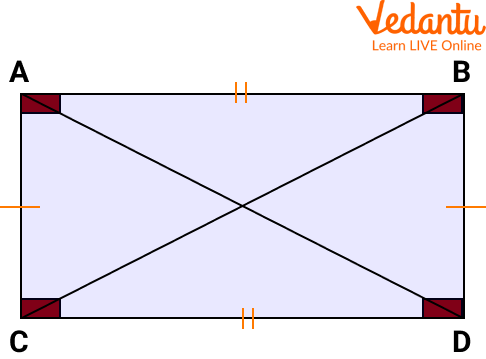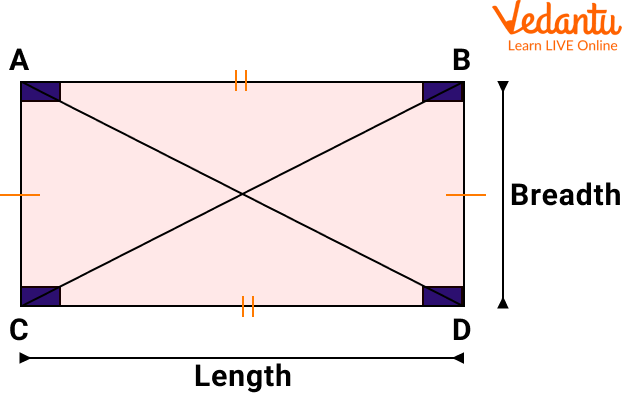Courses
Courses for Kids
Free study material
Offline Centres
More

# Perimeter and Area of a RectangleLast updated date: 02nd Dec 2023
Total views: 97.2k
Views today: 0.97kLet's imagine that you have a rectangular yard, and you want to fence it to protect it from street animals and you also want to decorate it with beautiful grass and flowers. So now you need to calculate its area and perimeter. After reading this question, you might wonder what is the rectangle. Here, we will learn about it by using examples of the perimeter and area of a rectangle. We will complete all this learning, keeping in mind the geometrical properties of a rectangle. So let's get started.

## What is a Rectangle with a Rectangle Diagram?

The rectangle is a closed quadrilateral, having four sides and four angles. The opposite sides of a rectangle are equal and parallel to each other. All the angles are equal and of $90^{\circ}$. The rectangle diagram is given below:Rectangle diagram

## Geometrical Properties of a Rectangle

• A rectangle is a quadrilateral.

• The opposite sides are parallel and equal to each other.

• Each interior angle is equal to $90^{\circ}$.

• The sum of all the interior angles is $360^{\circ}$.

• Both diagonals have the same length.

• The diagonals bisect each other.

## The Perimeter of a Rectangle

The perimeter of any shape is the total distance around its sides. It is defined as the length of the boundary or the outline of any shape. Remember one thing, perimeter changes as the shape and measure of the dimensions change.

The perimeter of a rectangle is defined as the total distance around the rectangle. For example, Let's imagine that there is a rectangle which is made with wire whose length is l. The same wire can be reused to make a triangle. Considering that all sides are equal in length. The perimeter diagram is given below:Perimeter Diagram

Rather than adding every side, we have some formula for calculating the perimeter of a rectangle. As the opposite sides of a rectangle are equal, its perimeter is given by length + breadth + length + breadth, which implies 2 (length + breadth). Thus, the formula to calculate the perimeter of the rectangle is:

$\mathrm{P}=2 \times(\mathrm{l}+\mathrm{b})$, where $\mathrm{I}$ is the length and $\mathrm{b}$ is the breadth of the rectangle.

## Area of a Rectangle

The area of any shape can be defined as the space required by it to fit the number of square units. The area of a rectangle is defined as the space occupied by it. It is calculated by using the dimensions of the rectangle. The area of a rectangle is given as the product of its length and breadth, i.e.

$A=L \times B$, where $L$ refers to the length and $B$ refers to the breadth of a rectangle.

Application of the Perimeter and the Area in Daily Life

• We need an area to calculate how much carpet is used to cover the floor.

• We need a perimeter to calculate how much wire is required for the fencing.

• While making swimming pools we need both perimeter and area to know the required dimension will fit in the area or not.

• In farming, we use the area to calculate how much grain we can grow on the given land.

## Solved Examples of the Perimeter and Area of a Rectangle

Q1. Find the perimeter of the figure shown below.Perimeter Diagram

Ans: The above figure shows that the rectangle is 9 inches in length and 3 inches in breadth. Here, $\mathrm{I}=9$ and $\mathrm{b}=3$

The perimeter of the rectangle $=2(l+b)$

$=2(3+9)$

$=2(12)$

$=24 \text { inches }$

Therefore, the perimeter of a rectangle is 24 inches.

Q2. Find the area of the figure which is given below.Rectangle Diagram

Ans: The above figure shows that the rectangle has a $5 \mathrm{~cm}$ length and $4 \mathrm{~cm}$ breadth.

Here, $l=5$ and $b=4$

Area of rectangle $=$ length $x$ breadth

$=5 \times 4$

$=20 \mathrm{~cm}^2$

Therefore, the area of a rectangle is $20 \mathrm{~cm}^2$.

## Practice Questions

Q1. Find the perimeter and area of the rectangle of length 17 cm and a breadth of 13 cm.

Ans: Perimeter $=60 \mathrm{~cm}$

Area $=221 \mathrm{~cm}^2$

Q2. Find the perimeter of a rectangle whose length and width are 20 cm and 9 cm respectively.

Ans: Perimeter = 58 cm

Q3. A rectangular yard has a length equal to 10 cm and a perimeter equal to 60 cm. Find its width.

Ans: Width = 20 cm

## Summary

So in this article, we have learned about the perimeter and area of a rectangle in a very enjoyable and learnable way. We learned that it only sounds tricky but it becomes very easy if paid a little attention. In this article, we have learned about the definitions of rectangle, perimeter, and area, along with the rectangle diagram and the perimeter diagram. We also studied the formula to calculate the area and perimeter of a rectangle, using some examples and practice problems.

## FAQs on Perimeter and Area of a Rectangle

1. What is the formula for calculating the perimeter of a circle?

The perimeter of a circle is also called the circumference of the circle. Its formula is given by $2 \pi r$.

2. Define the area of a square.

The area of a square is defined as the total space occupied by the square. The formula for calculating the area of the square is given by the square of its side, i.e. area of square $=a^2$, where $a$ is the side of a square.

3. What are the two standard units of measurement of the area?

Two standard units generally used for measuring the area of a geometrical figure are square centimetres and square metres, also written as $\mathrm{cm}^2$ and $\mathrm{m}^2$.